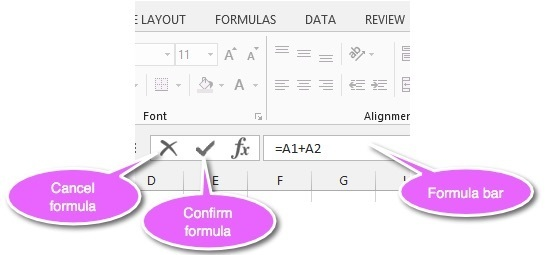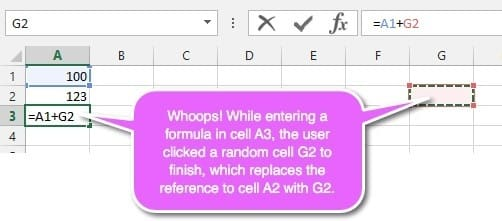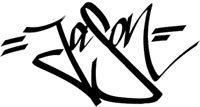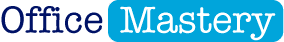# Excel Formulas Quick Start Guide: Get started today!

AUTHOR:  JASON MORRELLminutes remainingAre you struggling to get started with Excel formulas?

In this post I walk you through the process of building formulas, step-by-step. I’ll show you the proper way to create Excel formulas so you don’t get errors and you’ll discover some of the pitfalls you need to avoid.

So, to kick things off …

## 1.  What is a formula?

Put simply, a formula is something that does something with existing data (e.g. add numbers) or analyses data in some way.

• If you have 2 numbers and you want to add them together, you create a formula.
• If you want to work out a percentage of a number, you create a formula.
• If you want to pick out the largest number in a list, you use a formula.

Do you remember all the stuff you learnt back in maths class at school?

Did you just shudder?

Well, luckily you don’t need to remember 99% of your high school maths to use Excel formulas (phew!) but the very simplest things you learnt, even at primary school, like add, subtract, multiply and divide are important because you use them a lot.

The absolute most basic thing you’re likely to do in Excel is total up some numbers. It doesn’t matter if you’re a complete beginner or an Excel mastermind, you still need to add stuff up sometimes, right?

## 2. The 4 essential building blocks of formulas

There are 4 operations which you’ll need.

• Add (a.k.a. plus, sum).
• Subtract (a.k.a. minus, take away)
• Multiply (a.k.a. times by)
• Divide

The symbol for add and subtract are straight forward. You use the ‘+’ and ’-‘.

But be careful. On a regular keyboard, you get the ’+’ by holding down Shift, but to get the ‘-’ you simply press the ’-‘ key. If you accidentally press Shift and ’-’ you’ll get an underscore character (_).

The symbol for multiply is ‘*’ (asterisk). Not ‘x’. Press Shift 8 on a regular keyboard.

The symbol for divide is ‘/’. There is no regular divide symbol on a computer keyboard.

If you have an extended keyboard, all the mathematical operators just mentioned (+, -, * and /) are situated around the edge of the number padFigure 1: The number keypad on an extended keyboard has all the mathematical operators around the edge

## 3. What you need to know about Excel formulas

Excel formulas can use a mixture of

• Constants (e.g. 5)
• Cell references (e.g. A3)
• Cell ranges (e.g. A1:E5)

IMPORTANT: Every formula must begin with a ‘=’.

If you were to select a cell and type 2+2, then press Enter, it simply displays 2+2.

However, when you start with ‘=’, this is a trigger that tells Excel to calculate an answer. So =2+2 would give an answer of 4.

You can also refer to cells when creating formulas. For example, =C1+C3 would calculate the total of the numbers in cells C1 and C3.

Here are some examples of simple formulas:

## 4. How to ENTER Excel formulas

Either of these 2 methods can be used to enter a formula.

Method 1: Left-click a cell then type the formula directly into the cell.

Method 2: Left-click a cell then type the formula into the formula bar.

The formula bar is the large white box located between the toolbars and the column headers and to the right of the fx icon.Figure 02: The formula bar

Method 3: To finish a formula, press Enter or left-click the tick to the left of the formula bar ...

... or to cancel a formula (or changes to a formula), press Escape or left-click the cross to the left of the formula bar.

## 5. How to EDIT Excel formulas

Any of the following techniques can be used to edit the contents of a cell.

• Single-left-click the formula cell then click in the formula bar, make your changes and press Enter.
• Double-left-click the formula cell, make your changes in the cell or the formula bar and press Enter.
• Single-left-click the formula cell and press F2 to display the formula in the cell itself. Make your changes then press Enter.

## 6. What to watch out for when creating formulas

### #1 Accidentally using ‘=’ instead of ‘+’

The ‘+’ is on the same key as ‘=’ (on a regular keyboard).

If you type

###### =2+2

you get an answer of TRUE.

Excel compares 2 against 2 and asks the question “Are these 2 values the same?” In this case they are.

The formula

###### =2+3

would give an answer of FALSE because 2 does not equal 3.

Down the track, you can use this technique to your advantage to compare the values in 2 cells (e.g. =A1=A2) to see if they match (e.g. 2 totals).

### #2 Not completing the formula

If you create formulas that add up, let’s say, A1 and A2, you should always press Enter to complete the formula before moving to another cell.

Many people write a formula, let’s say

###### =A1+A2

then instead of pressing ENTER to lock in the formula, instead click a random cell to finish (let’s say, G2). What happens is that the formula now reads

###### =A1+G2

because A2 has been replaced by G2.

Always press Enter to finish your formula, then move onto the next thing.Figure 03: Always finish a formula by pressing Enter

### #3 Typing cell references rather that clicking on cell(s)

When you create a formula that uses cell references, always click on the cell reference(s) you need.

For example, to create the formula A1+A2 you should:

1.      Type ‘=’.

2.     Left-click cell A1.

3.     Type ‘+’.

4.     Left-click cell A2.

5.     Press Enter.

Too many people type cell references directly into a formula. This creates two ways for errors to creep in.

1.      Firstly, you may mis-read the cell-reference.

2.     Secondly, you might mis-type the cell reference.

3.     Or both.

So, minimise the possibility of errors by clicking the cell(s) you wish to use, as described above.

## 7. Watch the video (over the shoulder demo)## 8. What next?

In part 2, I’ll show you how to use formula functions to make life easier, especially when you are working with lots of numbers.

Please feel free to post any questions you have into the comments below. And before you leave this page, I want to give you one quick little action item!

ACTION ITEM: Comment below with ONE thing you picked up from this post and share how it has helped, or will help, you. It's good to share your successes because it encourages others and provides an instant boost.

Enjoy the rest of your day!Jason Morrell

Jason loves to simplify the hard stuff, cut the fluff and share what actually works. Things that make a difference. Things that slash hours from your daily work tasks. He runs a software training business in Queensland, Australia, lives on the Gold Coast with his wife and 4 kids and often talks about himself in the third person!

SHARE

POPULAR EXCEL POSTS

Excel screen: A beginners tour

How many row and columns does Excel have?

Formulas quick start guide

3 ways to calculate an average in Excel

How to calculate percentages

3 places to find help in Excel

14 easy ways to make your spreadsheet look fantastic

Smart ways to resize multiple columns at once

Excel's COUNT functions

«« SEE ALL EXCEL POSTS »»Master Microsoft Office
in 30 days or less

more from## How to fix formulas: 21 Fatal Formula Fails (and how to avoid them)

How to fix formulas: 21 Fatal Formula Fails (and how to avoid them)

## How to master the VLOOKUP formula in Excel

How to master the VLOOKUP formula in Excel

## Budgeting 101: Your Easy Guide to Creating a Business Budget in Excel

Budgeting 101: Your Easy Guide to Creating a Business Budget in Excel

## 2 Ways to Build a Dynamic Microsoft Word Index Page

2 Ways to Build a Dynamic Microsoft Word Index Page

JOIN THE DISCUSSION

{"email":"Email address invalid","url":"Website address invalid","required":"Required field missing"}